Summary alternating current is constantly changing in amplitude and direction. ADVANTAGES AND DISADVANTAGES OF AC AND DC - Direct current has several disadvantages compared to alternating current. ">Custom SearchSUMMARY Before going on to chapter 2, read the following summary of the material in chapter 1. This summary will reinforce what you have already learned. DC AND AC - Direct current flows in one direction only, while alternating current is constantly changing in amplitude and direction. ADVANTAGES AND DISADVANTAGES OF AC AND DC - Direct current has several disadvantages compared to alternating current. Direct current, for example, must be generated at the voltage level required by the load. Alternating current, however, can be generated at a high level and stepped down at the consumer end (through the use of a transformer) to whatever voltage level is required by the load. Since power in a dc system must be transmitted at low voltage and high current levels, the I2R power loss becomes a problem in the dc system. Since power in an ac system can be transmitted at a high voltage level and a low current level, the I2R power loss in the ac system is much less than that in the dc system. VOLTAGE WAVEFORMS - The waveform of voltage or current is a graphical picture of changes in voltage or current values over a period of time.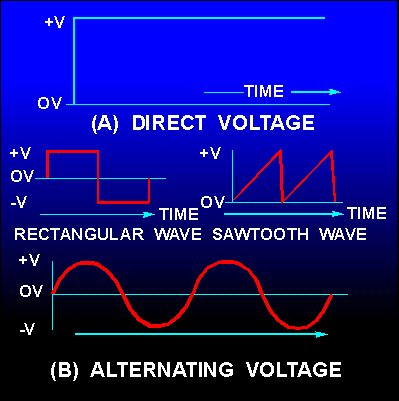ELECTROMAGNETISM - When a compass is placed in the vicinity of a current-carrying conductor, the needle aligns itself at right angles to the conductor. The north pole of the compass indicates the direction of the magnetic field produced by the current. By knowing the direction of current, you can use the left-hand rule for conductors to determine the direction of the magnetic lines of force.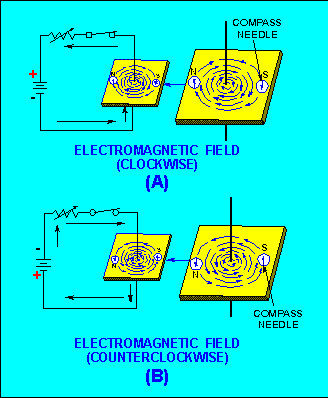Arrows are generally used in electrical diagrams to indicate the direction of current in a wire. A cross (+) on the end of a cross-sectional view of a wire indicates that current is flowing away from you, while a dot (·) indicates that current is flowing toward you. When two adjacent parallel conductors carry current in the same direction, the magnetic fields around the conductors aid each other. When the currents in the two conductors flow in opposite directions, the fields around the conductors oppose each other.MAGNETIC FIELD OF A COIL - When wire is wound around a core, it forms a COIL. The magnetic fields produced when current flows in the coil combine. The combined influence of all of the fields around the turns produce a two-pole field similar to that of a simple bar magnet. When the direction of current in the coil is reversed, the polarity of the two-pole field of the coil is reversed. The strength of the magnetic field of the coil is dependent upon: The number of turns of the wire in the coil. The amount of current in the coil. The ratio of the coil length to the coil width. The type of material in the core. BASIC AC GENERATION - When a conductor is in a magnetic field and either the field or the conductor moves, an emf (voltage) is induced in the conductor. This effect is called electromagnetic induction. A loop of wire rotating in a magnetic field produces a voltage which constantly changes in amplitude and direction. The waveform produced is called a sine wave and is a graphical picture of alternating current (ac). One complete revolution (360°) of the conductor produces one cycle of ac. The cycle is composed of two alternations: a positive alternation and a negative alternation. One cycle of ac in one second is equal to 1 hertz (1 Hz).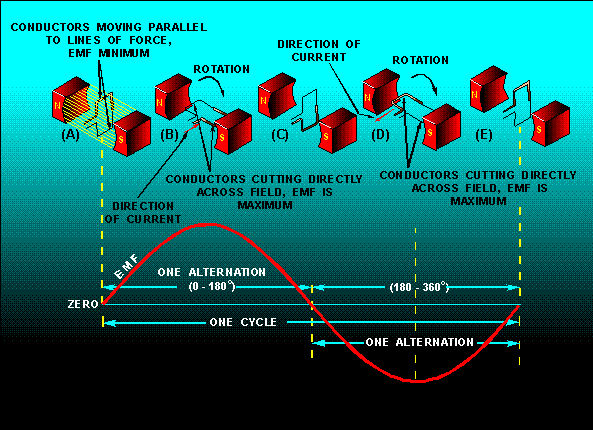FREQUENCY - The number of cycles of ac per second is referred to as the FREQUENCY. AC frequency is measured in hertz. Most ac equipment is rated by frequency as well as by voltage and current. PERIOD - The time required to complete one cycle of a waveform is called the PERIOD OF THE WAVE. Each ac sine wave is composed of two alternations. The alternation which occurs during the time the sine wave is positive is called the positive alternation. The alternation which occurs during the time the sine wave is negative is called the negative alternation. In each cycle of sine wave, the two alternations are identical in size and shape, but opposite in polarity. The period of a sine wave is inversely proportional to the frequency; e.g., the higher the frequency, the shorter the period. The mathematical relationships between time and frequency are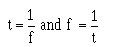WAVELENGTH - The period of a sine wave is defined as the time it takes to complete one cycle. The distance the waveform covers during this period is referred to as the wavelength. Wavelength is indicated by lambda (λ) and is measured from a point on a given waveform (sine wave) to the corresponding point on the next waveform.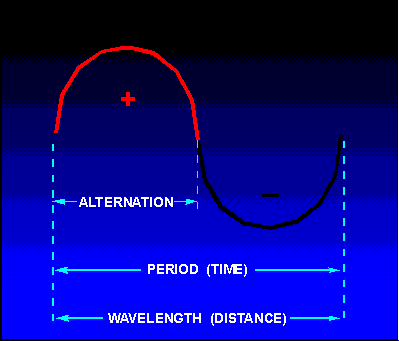PEAK AND PEAK-TO-PEAK VALUES - The maximum value reached during one alternation of a sine wave is the peak value. The maximum reached during the positive alternation to the maximum value reached during the negative alternation is the peak-to-peak value. The peak-to-peak value is twice the peak value.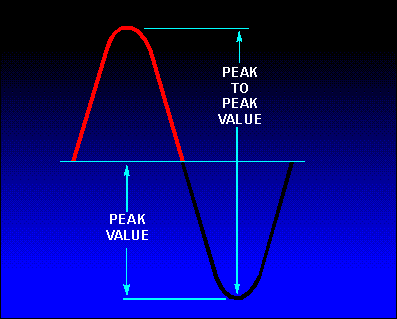INSTANTANEOUS VALUE - The instantaneous value of a sine wave of alternating voltage or current is the value of voltage or current at one particular instant of time. There are an infinite number of instantaneous values between zero and the peak value. AVERAGE VALUE - The average value of a sine wave of voltage or current is the average of all the instantaneous values during one alternation. The average value is equal to 0.636 of the peak value. The formulas for average voltage and average current are: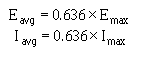Remember: The average value (Eavg or I avg) is for one alternation only. The average value of a complete sine wave is zero. EFFECTIVE VALUE - The effective value of an alternating current or voltage is the value of alternating current or voltage that produces the same amount of heat in a resistive component that would be produced in the same component by a direct current or voltage of the same value. The effective value of a sine wave is equal to 0.707 times the peak value. The effective value is also called the root mean square or rms value. The term rms value is used to describe the process of determining the effective value of a sine wave by using the instantaneous value of voltage or current. You can find the rms value of a current or voltage by taking equally spaced instantaneous values on the sine wave and extracting the square root of the average of the sum of the instantaneous values. This is where the term "Root-Mean-Square" (rms) value comes from. The formulas for effective and maximum values of voltage and current are:SINE WAVES IN PHASE - When two sine waves are exactly in step with each other, they are said to be in phase. To be in phase, both sine waves must go through their minimum and maximum points at the same time and in the same direction.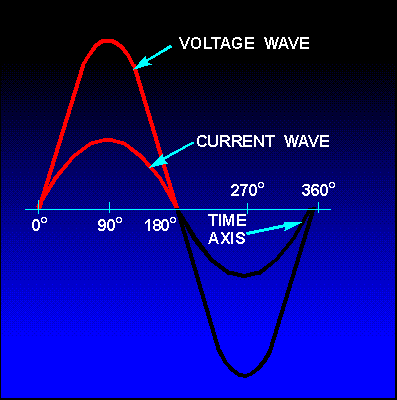SINE WAVES OUT OF PHASE - When two sine waves go through their minimum and maximum points at different times, a phase difference exists between them. The two waves are said to be out of phase with each other. To describe this phase difference, the terms lead and lag are used. The wave that reaches its minimum (or maximum) value first is said to lead the other wave. The term lag is used to describe the wave that reaches its minimum (or maximum) value some time after the first wave does. When a sine wave is described as leading or lagging, the difference in degrees is usually stated. For example, wave E1 leads wave E 2 by 90°, or wave E2 lags wave E 1 by 90°. Remember: Two sine waves can differ by any number of degrees except 0° and 360°. Two sine waves that differ by 0° or by 360° are considered to be in phase. Two sine waves that are opposite in polarity and that differ by 180° are said to be out of phase, even though they go through their minimum and maximum points at the same time.OHM'S LAW IN AC CIRCUIT - All dc rules and laws apply to an ac circuit that contains only resistance. The important point to remember is: Do not mix ac values. Ohm's Law formulas for ac circuits are given below: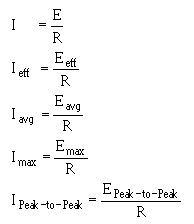Integrated Publishing, Inc. - A (SDVOSB) Service Disabled Veteran Owned Small Business